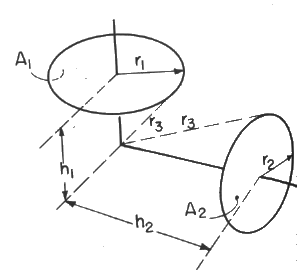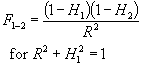# SECTION C Factors From Finite Areas to Finite Areas

## C-43: Nonintersecting disks with intersecting axes; axes intersect between disks, and disks can be inscribed in sphere of radius r3 (i.e., r12+h12=r22+h22=r32).Definitions: H = h/r3; R = r1/r3

Governing equation:To download a copy of this equation in MS Word 97 Format, click HERE.